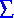# Algorithmic (a.k.a. Computational) Number Theory: Tables, Links, etc.

Tables of solutions and other information concerning Diophantine equations [equations where the variables are constrained to be integers or rational numbers]:
• Trinomials with unusual Galois groups (x5-5x2-3,  x5-5x2+15,  x7-7x+3,  x8+324x+567, etc.)
• (Supporting computational data for Nils Bruin's theorem here)
• Elliptic curves of large rank and small conductor (arXiv preprint; joint work with Mark Watkins; to appear in the proceedings of ANTS-VI (2004)): Elliptic curves over Q of given rank r up to 11 of minimal conductor or discriminant known; these are new records for each r in [6,11]. We describe the search method tabulate the top 5 (bottom 5?) such curves we found for r in [5,11] for low conductor, and for r in [5,10] for low discriminant.
• Data and results concerning the elliptic curves ny2=x3-x arising in the “congruent number” problem:
• Transparencies for ANTS-V lecture, in PS and PDF (added 7/2002)
• All |n|=8k+7<106 for which the curve has analytic rank at least 3, listed and used;
• The same list for |n|=8k+5<106, under an additional conjecture;
• Ditto for |n|=8k+6<106. Combining these three, we conclude that every “congruent number” curve of sign -1 with |n|<106 has positive rank.
• More rank-3 twists in the same congruence classes: all |n|=8k+7<107 such that the curve has rank at least 3 assuming the conjecture of Birch and Swinnerton-Dyer; all |n|=8k+5<107 such that the curve has rank at least 3 under the assumptions of BSD and generalized Gross-Zagier; and similarly for |n|=8k+6<107, where curiously there are significantly more such |n| with k even than there are with k odd, and the combined list is much longer than either the 8k+5 or 8k+7 list.
• Elliptic curves x3 + y3 = k of high rank (arXiv preprint; joint work with Nicholas Rogers, based on his doctoral thesis; to appear in the proceedings of ANTS-VI (2004)): The first examples of elliptic curves Ek: x3 + y3 = k of ranks 8, 9, 10, 11 over Q, and thus also the first examples of elliptic curves xy(x+y)=k with a rational 3-torsion point and rank as high as 11 (the previous record was 8). We also discuss the problem of finding the minimal curve Ek of a given rank, in the sense of both |k| and the conductor of Ek, and we give some new results in this direction.
• Rational points on the twisted Fermat cubic curves x3 + y3 = pf z3 (f=1, f=2) for certain primes p (updated 11/2002, to report on directly-computed values of L'(E,1) and on new experimental data for p=9k+8 with f=1; and again 4/2003 and 9/2004, to include (p,f)=(9k+8,2) data)
• Orbit representatives of the 218044170240 minimal vectors in the rank-128 Mordell-Weil lattice of y2+y=x3+t65+a6 in characteristic 2 (for the context, see this preprint, now published in the journal Experimental Mathematics [10 (2001) #3, 467-473]).
• Data concerning Hall's conjecture: small nonzero values of |x3-y2|
• update 6/2003: Calvo, Sáez and Herranz propose another search algorithm, which has been used to recover the all the solutions in my table and a dozen new ones; see this page for the list and some information about their new algorithm. I do not know how the two algorithms are related; I suspect, but have not proved, that they turn out to be quite close, but that the Calvo-Sáez-Herranz approach finds more examples by searching in families likely to contain them, with the downside that one cannot guarantee that it will find all solutions up to some bound on x,y.
• My 1988 construction of elliptic curves y2=x3+ax+b with a large integral point (added 6/2003)
• Fermat “near-misses”: small values of |xn + yn - zn| (n=4,5,...,20; for n=3 see Dan Bernstein's tables [added 6/2002: and also H.Mishima's page on the n=x3+y3+z3 problem])
• Elliptic curves over Q with nontorsion rational points of low canonical height (added 6/2002)
• For some results and questions on the corresponding question for curves over P1(C) etc., see my lecture transparencies in PS or PDF; the linear combinations of second Bernoulli functions that I used are plotted for x in [0,1/2] (a fundamental domain for the infinite dihedral symmetry group) in PS and GIF for sigma=6, and again for sigma=4 (PS, GIF -- for some reason I can't convert these .ps plots to .pdf).
• An elliptic curve y2=x3-Dx of rank 13 (updated 5/2002: also rank 14)
• Examples of simple genus-2 Jacobians with rational torsion points of high order, and a few other new amusing examples of curves of genus 2 over Q (updated 6/2002: more rank-1 cases)
• Explicit Hecke correspondences for use in the construction of towers of modular curves:
• Explicit complete parametrization of the cubic Fermat surface w3+x3+y3+z3=0 by homogeneous cubics in three variables (thanks to Dave Rusin for keeping my original posting to rec.puzzles and sci.math of these formulas at his known-math archives)

Links (see also this list at the Number Theory Web):

Algorithmic number theory? What's that?Back to general math page